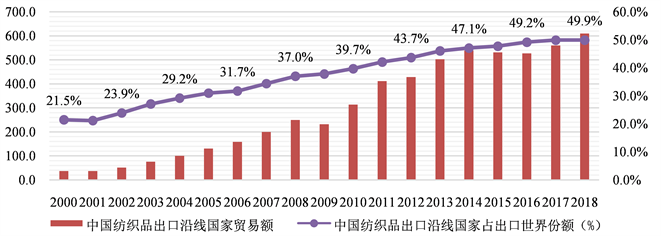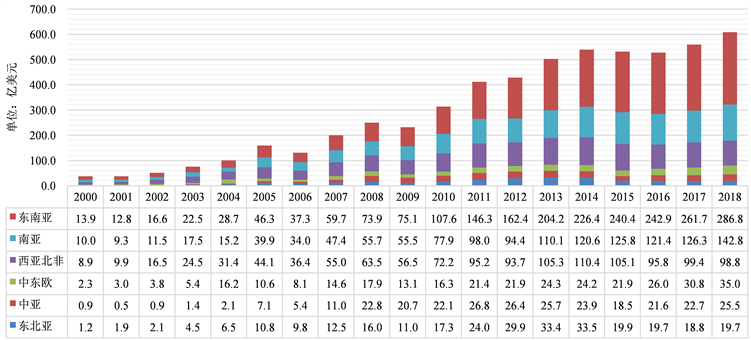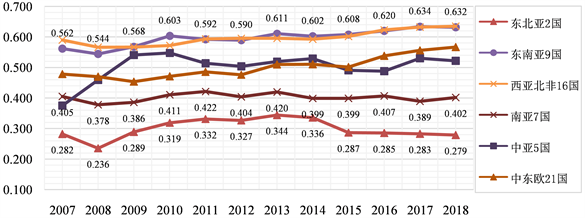﻿ 中国对“一带一路”沿线六大区域纺织出口效率研究

# 中国对“一带一路”沿线六大区域纺织出口效率研究Research on Textile Export Efficiency from China to Six Regions along the “One Belt and One Road”

Abstract: 2020 is the seventh year of the One Belt and One Road initiative, and the cooperation strategy be-tween China with countries along the One Belt and One Road will continue to advance during the seven years. Chinese textile industry is facing the pressure from decreasing traditional advantages and the internal and external adverse environment. For the textile industry continuing to play its positive role in the national economy, this article starts the research at the base of present situation of Chinese textile export from 2007 to 2018, six regions along the 60 countries as the research ob-ject, by constructing the stochastic frontier model of gravity and trade non-efficiency model, to cal-culate export efficiency of textile and analyze the main influencing factors of trade efficiency, and then puts forward some effective suggestions.

1. 引言

2. 纺织品出口现状

2.1. 出口贸易额Figure 1. China’s textile export to “One Belt and One Road” countries

2.2. 六大区域出口现状Figure 2. The export volume of Chinese textiles to the six regions of “One Belt and One Road”

3. 模型、变量与数据

3.1. 随机前沿引力模型

${T}_{ijt}=f\left({X}_{ijt},\beta \right)\mathrm{exp}\left({\nu }_{ijt}\right)\mathrm{exp}\left(-{\mu }_{ijt}\right),\text{\hspace{0.17em}}{\mu }_{ijt}\ge 0$ (1)

${T}_{ijt}^{*}=f\left({X}_{ijt},\beta \right)\mathrm{exp}\left({\nu }_{ijt}\right)$ (2)

$T{E}_{ijt}={T}_{ijt}/{T}_{ijt}^{\ast }=\mathrm{exp}\left(-{\mu }_{ijt}\right),\text{\hspace{0.17em}}T{E}_{ijt}\in \left(0,1\right]$ (3)

${\mu }_{it}={\eta }_{it}{\mu }_{i}=\left\{\mathrm{exp}\left[-\eta \left(t-T\right)\right]\right\}{\mu }_{t},\text{\hspace{0.17em}}\text{\hspace{0.17em}}t\in T\left(i\right);\text{\hspace{0.17em}}\text{\hspace{0.17em}}i=1,2,\cdots ,N$ (4)

${\mu }_{it}=h\left({z}_{it},\alpha \right)+{\omega }_{it}=\alpha {z}_{it}+{\omega }_{it}$ (5)

$\mathrm{ln}{T}_{ijt}=\mathrm{ln}f\left({X}_{ijt},\beta \right)+{\nu }_{ijt}-\left\{\mathrm{exp}\left[-\eta \left(t-T\right)\right]\right\}{\mu }_{ij}$ (6)

3.2. 模型设定与变量

3.2.1. 时变随机前沿引力模型的设定

$\begin{array}{c}\mathrm{ln}{T}_{ijt}={\beta }_{0}+{\beta }_{1}\mathrm{ln}GD{P}_{it}+{\beta }_{2}\mathrm{ln}GD{P}_{jt}+{\beta }_{3}\mathrm{ln}Po{p}_{it}+{\beta }_{4}\mathrm{ln}Po{p}_{jt}\\ \text{\hspace{0.17em}}\text{\hspace{0.17em}}+{\beta }_{5}\mathrm{ln}Di{s}_{ij}+{\beta }_{5}Bo{r}_{ij}+{\beta }_{6}L{L}_{ij}+{\nu }_{ijt}-{\mu }_{ijt}\end{array}$ (7)Table 1. System resulting data of standard experiment

3.2.2. 贸易非效率模型的设定

$\begin{array}{c}{\mu }_{ijt}={\alpha }_{0}+{\alpha }_{1}FT{A}_{ij}+{\alpha }_{2}P{S}_{jt}+{\alpha }_{3}G{E}_{jt}+{\alpha }_{4}Ex{R}_{it}+{\alpha }_{5}T{F}_{jt}\\ \text{\hspace{0.17em}}\text{\hspace{0.17em}}+{\alpha }_{6}LP{I}_{jt}+{\alpha }_{7}Ta{x}_{jt}+{\omega }_{ijt}\end{array}$ (8)Table 2. Description of trade non-efficiency model variables and data sources

3.3. 样本说明

4. 实证结果分析

4.1. 模型设定的检验

4.1.1. 时变随机前沿模型的检验Table 4. Likelihood ratio test results of SFA

Table 5. Regression results of TIM and TAM

4.1.2. 贸易非效率模型的假设检验Table 6. Test results of trade non-efficiency model variable selection

$\begin{array}{l}\mathrm{ln}{T}_{ijt}={\beta }_{0}+{\beta }_{1}\mathrm{ln}GD{P}_{it}+{\beta }_{2}\mathrm{ln}GD{P}_{jt}+{\beta }_{3}\mathrm{ln}Po{p}_{it}+{\beta }_{4}\mathrm{ln}Po{p}_{jt}\\ \text{\hspace{0.17em}}\text{\hspace{0.17em}}\text{\hspace{0.17em}}\text{\hspace{0.17em}}\text{\hspace{0.17em}}\text{\hspace{0.17em}}\text{\hspace{0.17em}}\text{\hspace{0.17em}}\text{\hspace{0.17em}}\text{\hspace{0.17em}}+{\beta }_{5}\mathrm{ln}Di{s}_{ij}+{\beta }_{6}Bo{r}_{ij}+{\beta }_{7}L{L}_{ij}+{\nu }_{ijt}-{\mu }_{ijt}\end{array}$

${\mu }_{ijt}={\alpha }_{0}+{\alpha }_{1}FT{A}_{ij}+{\alpha }_{2}P{S}_{jt}+{\alpha }_{3}G{E}_{jt}+{\alpha }_{4}Ex{R}_{it}+{\alpha }_{5}T{F}_{jt}+{\alpha }_{6}LP{I}_{jt}+{\omega }_{ijt}$ (9)

4.2. 实证模型回归结果分析Table 7. “One-step method” model regression results

4.2.1. 随机前沿引力模型结果分析

4.2.2. 贸易非效率模型结果分析

4.3. 出口效率分析Figure 3. China’s textile export efficiency to six regions along the Belt and Road from 2007 to 2018

5. 相关建议

5.1. 提高国家经济发展水平

5.2. 加强双边自贸协定建设

5.3. 适度调控人民币汇率

5.4. 提升基础设施建设水平

 盛斌, 廖明中. 中国的贸易流量与出口潜力: 引力模型的研究[J]. 世界经济, 2004(2): 3-12.

 张剑光, 张鹏. 中国与“一带一路”国家的贸易效率与影响因素研究[J]. 国际经贸探索, 2017, 33(8): 4-23.

 Battese, T. and Coelli, J. (1992) Frontier Productions Function, Technical Efficiency and Panel Data: With Application to Paddy Farmers in India. Journal of Productivity Analysis, 3, 153-169.
https://doi.org/10.1007/BF00158774

 Battese, G.E. and Coelli, T.J. (1995) A Model for Technical Inefficiency Effects in a Stochastic Frontier Production Function for Panel Da-ta. Empirical Economics, 20, 325-332.
https://doi.org/10.1007/BF01205442

Top